Class VI - Mathematics

# NCERT Solutions: Chapter - 3 Playing with Numbers

Other Chapters

Page 50

Exercise 3.1

Q1. Write all the factors of the following numbers:
(a) 24 (b) 15 (c) 21
(d) 27 (e) 12 (f) 20
(g) 18 (h) 23 (i) 36

A factor of a number is an exact divisor of that number.
(a) 24
24 = 1 × 24
24 = 2 × 12
24 = 3 × 8
24 = 4 × 6
∴ Factors of 24 are 1, 2, 3, 4, 6, 8, 12 and 24.

(b) 15
15 = 1 × 15
15 = 3 × 5
∴ Factors of 15 are 1, 3, 5 and 15.

(c) 21
21 = 1 × 21
21 = 3 × 7
∴ Factors of 21 are 1, 3, 7 and 21.

(d) 27

27 = 1 × 27

27 = 3 × 9

∴ Factors of 27 are 1, 3, 9 and 27.

(e) 12
12 = 1 × 12
12 = 2 × 6
12 = 3 × 4
∴ Factors of 12 are 1, 2, 3, 4, 6 and 12.

(f) 20
20 = 1 × 20
20 = 2 × 10
20 = 4 × 5
∴ Factors of 20 are 1, 2, 4, 5, 10 and 20.

(g) 18
18 = 1 × 18
18 = 2 × 9
18 = 3 × 6
∴ Factors of 18 are 1, 2, 3, 6, 9 and 18.

(h) 23
23 = 1 × 23
∴ Factors of 23 are 1 and 23.

(i) 36
36 = 1 × 36
36 = 2 × 18
36 = 3 × 12
36 = 4 × 9
36 = 6 × 6
∴ Factors of 36 are 1, 2, 3, 4, 6, 9, 12, 18 and 36.

Q2. Write first five multiples of:
(a) 5 (b) 8 (c) 9

(a) 5
5 × 1 = 5
5 × 2 = 10
5 × 3 = 15
5 × 4 = 20
5 × 5 = 25
∴ First five multiples of 5 are 5, 10, 15, 20 and 25.

(b) 8
8 × 1 = 8
8 × 2 = 16
8 × 3 = 24
8 × 4 = 32
8 × 5 = 40
∴ First five multiples of 8 are 8, 16, 24, 32 and 40.

(c) 9
9 × 1 = 9
9 × 2 = 18
9 × 3 = 27
9 × 4 = 36
9 × 5 = 45
∴ First five multiples of 9 are 9, 18, 27, 36 and 45.

Q3. Match the items in column 1 with the items in column 2.

 Column 1 Column 2 (i) 35 (a) Multiple of 8 (ii) 15 (b) Multiple of 7 (iii) 16 (c) Multiple of 70 (iv) 20 (d) Factor of 30 (v) 25 (e) Factor of 50 (f) Factor of 20

 Column 1 Column 2 (i) 35 (b) Multiple of 7 (ii) 15 (d) Factor of 30 (iii) 16 (a) Multiple of 8 (iv) 20 (f) Factor of 20 (v) 25 (e) Factor of 50

Q4. Find all the multiples of 9 upto 100.

9 × 1 = 9
9 × 2 = 18
9 × 3 = 27
9 × 4 = 36
9 × 5 = 45
9 × 6 = 54
9 × 7 = 63
9 × 8 = 72
9 × 9 = 81
9 × 10 = 90
9 × 11 = 99
9 × 12 = 108
∴ All the multiples of 9 upto 100 are 9, 18, 27, 36, 45, 54, 63, 72, 81, 90 and 99.

Page 53

Exercise 3.2

Q1. What is the sum of any two (a) Odd numbers? (b) Even numbers?

(a) Odd numbers

• 3 + 7 = 10
• 5 + 9 = 14
The sum of any two odd numbers is an even number.
(b) Even numbers
• 2 + 8 = 10
• 4 + 14 = 18
The sum of any two even numbers is an even number.

Q2. State whether the following statements are True or False:
(a) The sum of three odd numbers is even.
(b) The sum of two odd numbers and one even number is even.
(c) The product of three odd numbers is odd.
(d) If an even number is divided by 2, the quotient is always odd.
(e) All prime numbers are odd.
(f) Prime numbers do not have any factors.
(g) Sum of two prime numbers is always even.
(h) 2 is the only even prime number.
(i) All even numbers are composite numbers.
(j) The product of two even numbers is always even.

(a) False, The sum of three odd numbers will always be odd. Suppose we take 3 odd numbers, say 3, 5 and 7, their sum will be 3 + 5 + 7 = 15 which is not an even number.
(b) True, The sum of any two odd numbers is even and the sum of any two even numbers is even. Suppose we take 2 odd numbers, say, 3, 5 and one even number, say 6, their sum will be 3 + 5 + 6 = 14 which is an even number.
(c) True, The product of any odd numbers is odd. Suppose we take 3 odd numbers, say 3, 5 and 7, their product will be 3 × 5 × 7 = 15 × 7 = 105, which is an odd number.
(d) False, If an even number is divided by 2, the quotient can be either odd or even, for example 8 + 2 = 4 (even) and 26 + 2 = 13 (odd).
(e) False, 2 which is an even number is the smallest prime number.
(f) False, The numbers other than 1 whose only factors are 1 and the number itself are called Prime numbers.
(g) False, This statement can only be true if neither of the two prime numbers that are added is 2. When 2 is not being considered than the sum of two prime numbers is always even.
(h) True, every prime number except 2 is odd.
(i) False, 2 which is an even number is the smallest prime number. Except 2, all even numbers are composite numbers.
(j) True, The product of two even numbers is always even, for example 2 × 4 = 8 (even) and 12 × 2 = 24 (even).

Q3. The numbers 13 and 31 are prime numbers. Both these numbers have same digits 1 and 3. Find such pairs of prime numbers upto 100.

(17, 71), (37, 73) (79, 97)

Q4. Write down separately the prime and composite numbers less than 20.

The numbers other than 1 whose only factors are 1 and the number itself are called Prime numbers. The prime numbers which are less than 20 are 2, 3, 5, 7, 11, 13, 17 and 19.
Numbers having more than two factors are called Composite numbers. The composite numbers which are less than 20 are 4, 6, 8, 9, 10, 12, 14, 15, 16 and 18.

Q5. What is the greatest prime number between 1 and 10?

The prime numbers between 1 and 10 are 2, 3, 5 and 7.
Therefore the greatest prime number between 1 and 10 will be 7.

Q6. Express the following as the sum of two odd primes.
(a) 44 (b) 36 (c) 24 (d) 18

(a) 44 = 3 + 41
(b) 36 = 5 + 31
(c) 24 = 7 + 17
(d) 18 = 5 + 13

Q7. Give three pairs of prime numbers whose difference is 2.
[Remark: Two prime numbers whose difference is 2 are called twin primes].

Two prime numbers whose difference is ‘2’ is also called as twin prime number or twin primes.
Three pairs of prime numbers whose difference is 2 are (5, 3), (7, 5) and (13, 11).
Other such twin primes can be: (19, 17), (43, 41), (73,71)

Q8. Which of the following numbers is Prime?
(a) 23 (b) 51 (c) 37 (d) 26

(a) The factors of 23 are 1 × 23 = 23
(b) The factors of 51 are 1 × 51 and 3 × 17 = 51
(c) The factors of 37 are 1 × 37 = 37
(d) The factors of 26 are 1 × 26 = 26 and 2 × 13 = 26
Prime numbers are numbers whose only factors are 1 and itself.
∴ Out of the given numbers only 23 and 37 are prime numbers.

Q9. Write seven consecutive composite numbers less than 100 so that there is no prime number between them.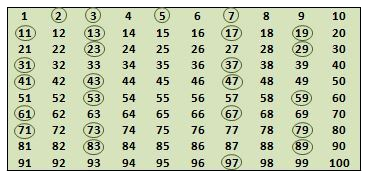The encircled numbers are prime numbers. The rest of the numbers, except 1, are composite numbers.
By observing the Sieve Method, we can see that seven consecutive numbers that do not have any prime number between them are 90, 91, 92, 93, 94, 95 and 96.

Q10. Express each of the following numbers as the sum of three odd primes:
(a) 21 (b) 31 (c) 53 (d) 61

(a) 21 = 3 + 5 + 13 or 3 + 7 + 11
(b) 31 = 5 + 7 + 19 or 7 + 11 + 13 or 3 + 5 + 23 or 3 + 11 + 17
(c) 53 = 3 + 21 + 29 or 3 + 13 + 37 or 5 + 11 + 37 or 13 + 17 + 23
(d) 61 = 13 + 17 + 31 or 7 + 13 + 41 or 3 + 17 + 41

Q11. Write five pairs of prime numbers less than 20 whose sum is divisible by 5.
(Hint: 3 + 7 = 10)

Prime numbers less than 20 are 2, 3, 5, 7, 11, 13, 17 and 19.
Pairs of prime numbers less than 20 whose sum is divisible by 5 are
(i) 2 + 3 = 5 [5 × 1 = 5, is divisible by 5]
(ii) 2 + 13 = 15 [5 × 3 = 15, is divisible by 5]
(iii) 3 + 7 = 10 [5 × 3 = 10, is divisible by 5]
(iv) 3 + 17 = 20 [5 × 4 = 20, is divisible by 5]
(v) 7 + 13 = 20 [5 × 4 = 20, is divisible by 5]
(vi) 11 + 19 = 30 [5 × 6 = 30, is divisible by 5]
(vii) 13 + 17 = 30 [5 × 6 = 30, is divisible by 5]

Q12. Fill in the blanks:
(a) A number which has only two factors is called a ______.
(b) A number which has more than two factors is called a ______.
(c) 1 is neither ______ nor ______.
(d) The smallest prime number is ______.
(e) The smallest composite number is _____.
(f) The smallest even number is _____.

(a) A number which has only two factors is called a prime number.
(b) A number which has more than two factors is called a composite number.
(c) 1 is neither prime nor composite.
(d) The smallest prime number is 2.
(e) The smallest composite number is 4.
(f) The smallest even number is 2.

Page 57

Exercise 3.3

Q1. Using divisibility tests, determine which of the following numbers are divisible by 2; by 3; by 4; by 5; by 6; by 8; by 9; by 10; by 11 (say, yes or no):

 Number Divisible by 2 3 4 5 6 8 9 10 11 128 990 1586 275 6686 639210 429714 2856 3060 406839

Tests for Divisibility of Numbers
Divisibility by 2: a number is divisible by 2 if it has any of the digits 0, 2, 4, 6 or 8 in its ones place.
Divisibility by 3: if the sum of the digits is a multiple of 3, then the number is divisible by 3.
Divisibility by 4: a number with 3 or more digits is divisible by 4 if the number formed by its last two digits (i.e. ones and tens) is divisible by 4.
Divisibility by 5: a number which has either 0 or 5 in its ones place is divisible by 5
Divisibility by 6: if a number is divisible by 2 and 3 both then it is divisible by 6 also.
Divisibility by 8: a number with 4 or more digits is divisible by 8, if the number formed by the last three digits is divisible by 8
Divisibility by 9: if the sum of the digits of a number is divisible by 9, then the number itself is divisible by 9.
Divisibility by 10: if a number has 0 in the ones place then it is divisible by 10.
Divisibility by 11: if the difference between the sum of the digits at odd places (from the right) and the sum of the digits at even places (from the right) of the number is either 0 or divisible by 11, then the number is divisible by 11.

 Number Divisible by 2 3 4 5 6 8 9 10 11 128 Yes No Yes No No Yes No No No 990 Yes Yes No Yes Yes No Yes Yes Yes 1586 Yes No No No No No No No No 275 No No No Yes No No No No Yes 6686 Yes No No No No No No No No 639210 Yes Yes No Yes Yes No No Yes Yes 429714 Yes Yes No No Yes No Yes No No 2856 Yes Yes Yes No Yes Yes No No No 3060 Yes Yes Yes Yes Yes No Yes Yes No 406839 No Yes No No No No No No No

Q2. Using divisibility tests, determine which of the following numbers are divisible by 4; by 8:
(a) 572 (b) 726352 (c) 5500 (d) 6000 (e) 12159 (f) 14560 (g) 21084 (h) 31795072 (i) 1700 (j) 2150

Divisibility by 4: a number with 3 or more digits is divisible by 4 if the number formed by its last two digits (i.e. ones and tens) is divisible by 4.
Divisibility by 8: a number with 4 or more digits is divisible by 8, if the number formed by the last three digits is divisible by 8

(a) 572
72 + 4 = 18, therefore the number is divisible by 4
572 is not divisible by 8 therefore the number is not divisible by 8

(b) 726352
52 + 4 = 13, therefore the number is divisible by 4
352 + 8 = 44, therefore the number is divisible by 8

(c) 5500
0 + 4 = 0, therefore the number is divisible by 4
500 is not divisible by 8 therefore the number is not divisible by 8

(d) 6000
0 + 4 = 0, therefore the number is divisible by 4
0 + 8 = 0, therefore the number is divisible by 8

(e) 12159
59 is not divisible by 4, therefore the number is not divisible by 4
159 is not divisible by 8 therefore the number is not divisible by 8

(f) 14560
60 + 4 = 15, therefore the number is divisible by 4
560 + 8 = 70, therefore the number is divisible by 8

(g) 21084

84 + 4 = 21, therefore the number is divisible by 4
84 is not divisible by 8 therefore the number is not divisible by 8

(h) 31795072
72 + 4 = 18, therefore the number is divisible by 4
72 + 8 = 9, therefore the number is divisible by 8

(i) 1700
0 + 4 = 0, therefore the number is divisible by 4
700 is not divisible by 8 therefore the number is not divisible by 8

(j) 2150

50 is not divisible by 4 therefore the number is not divisible by 4
150 is not divisible by 8 therefore the number is not divisible by 8

Q3. Using divisibility tests, determine which of following numbers are divisible by 6:
(a) 297144 (b) 1258 (c) 4335 (d) 61233 (e) 901352 (f) 438750 (g) 1790184 (h) 12583 (i) 639210 (j) 17852

Divisibility by 6: if a number is divisible by 2 and 3 both then it is divisible by 6 also.
(a) 297144
The number has 4 in its ones place so it is divisible by 2
The sum of the digits = 2 + 9 + 7 + 1 + 4 + 4 = 27, which is divisible by 3
Since the number is divisible by both 2 and 3, hence ‘297144’ is divisible by 6.

(b) 1258
The number has 8 in its ones place so it is divisible by 2
The sum of the digits = 1 + 2 + 5 + 8 = 16, which is not divisible by 3
Since the number is divisible by 2 but not 3, hence ‘1258’ is not divisible by 6.

(c) 4335
The number has 5 in its ones place so it is not divisible by 2
The sum of the digits = 4 + 3 + 3 + 5 = 15, which is divisible by 3
Since the number is divisible by 3 but not 2, hence ‘4335’ is not divisible by 6.

(d) 61233
The number has 3 in its ones place so it is not divisible by 2
The sum of the digits = 6 + 1 + 2 + 3 + 3 = 15, which is divisible by 3
Since the number is divisible by 3 but not 2, hence ‘61233’ is not divisible by 6.

(e) 901352
The number has 2 in its ones place so it is divisible by 2
The sum of the digits = 9 + 0 + 1 + 3 + 5 + 2 = 20, which is not divisible by 3
Since the number is divisible by 2 but not 3, hence ‘901352’ is not divisible by 6.

(f) 438750
The number has 0 in its ones place so it is divisible by 2
The sum of the digits = 4 + 3 + 8 + 7 + 5 + 0 = 27, which is divisible by 3
Since the number is divisible by both 2 and 3, hence ‘438750’ is divisible by 6.

(g) 1790184
The number has 4 in its ones place so it is divisible by 2
The sum of the digits = 1 + 7 + 9 + 0 + 1 + 8 + 4 = 30, which is divisible by 3
Since the number is divisible by both 2 and 3, hence ‘1790184’ is divisible by 6.

(h) 12583
The number has 3 in its ones place so it is not divisible by 2
The sum of the digits = 1 + 2 + 5 + 8 + 3 = 19, which is not divisible by 3
Since the number is not divisible by both 2 and 3, hence ‘12583’ is not divisible by 6.

(i) 639210
The number has 0 in its ones place so it is divisible by 2
The sum of the digits = 6 + 3 + 9 + 2 + 1 + 0 = 21, which is divisible by 3
Since the number is divisible by both 2 and 3, hence ‘639210’ is divisible by 6.

(j) 17852
The number has 2 in its ones place so it is divisible by 2
The sum of the digits = 1 + 7 + 8 + 5 + 2 = 23, which is not divisible by 3
Since the number is divisible by 2 but not 3, hence ‘17852’ is not divisible by 6.

Q4. Using divisibility tests, determine which of the following numbers are divisible by 11:
(a) 5445 (b) 10824 (c) 7138965 (d) 70169308 (e) 10000001 (f) 901153

Divisibility by 11: if the difference between the sum of the digits at odd places (from the right) and the sum of the digits at even places (from the right) of the number is either 0 or divisible by 11, then the number is divisible by 11.
(a) 5445
The sum of the digits in the odd places from the right = 5 + 4 = 9
The sum of the digits in the even places from the right = 4 + 5 = 9
Difference = 9 – 9 = 0
The number is divisible by 11.

(b) 10824
Sum of the digits in the odd places from the right = 4 + 8 + 1 = 13
Sum of the digits in the even places from the right = 2 + 0 = 2
Difference = 13 – 2 = 11
The number is divisible by 11.

(c) 7138965
Sum of the digits in the odd places from the right = 5 + 9 + 3 + 7 = 24
Sum of the digits in the even places from the right = 6 + 8 + 1 = 15
Difference = 24 – 15 = 9
The number is not divisible by 11.

(d) 70169308
Sum of the digits in the odd places from the right = 8 + 3 + 6 + 0 = 17
Sum of the digits in the even places from the right = 0 + 9 + 1 + 7 = 17
Difference = 17 – 17 = 0
The number is divisible by 11.

(e) 10000001
Sum of the digits in the odd places from the right = 1 + 0 + 0 + 0 = 1
Sum of the digits in the even places from the right = 0 + 0 + 0 + 1 = 1
Difference = 1 – 1 = 0
The number is divisible by 11.

(f) 901153
Sum of the digits in the odd places from the right = 3 + 1 + 0 = 4
Sum of the digits in the even places from the right = 5 + 1 + 9 = 15
Difference = 15 – 4 = 11
The number is divisible by 11.

Q5. Write the smallest digit and the greatest digit in the blank space of each of the following numbers so that the number formed is divisible by 3:
(a) __ 6724 (b) 4765 __ 2

Divisibility by 3: if the sum of the digits is a multiple of 3, then the number is divisible by 3.
(a) _6724
The sum of the digits = _ + 6 + 7 + 2 + 4 = 19
The smallest digit that can be added to 19 is 0.
19 + 0 = 19, is not a multiple of 3
19 + 1 = 20, is not a multiple of 3
19 + 2 = 21, is a multiple of 3
Therefore the smallest digit that should be in the blank space is 2.

The greatest digit that can be added is 9.
19 + 9 = 28, is not a multiple of 3
19 + 8 = 27, is a multiple of 3
Therefore the greatest digit in the blank space should be 8.

(b) 4765_2
The sum of the digits = 4 + 7 + 6 + 5 + _ + 2 = 24
The smallest digit that can be added to 24 is 0.
24 + 0 = 24, is a multiple of 3
Therefore the smallest digit that should be in the blank space is 0.

The greatest digit that can be added is 9.
24 + 9 = 33, is a multiple of 3
Therefore the greatest digit in the blank space should be 9.

Q6. Write a digit in the blank space of each of the following numbers so that the number formed is divisible by 11:
(a) 92 __ 389 (b) 8 __ 9484

Divisibility by 11: if the difference between the sum of the digits at odd places (from the right) and the sum of the digits at even places (from the right) of the number is either 0 or divisible by 11, then the number is divisible by 11.
(a) 92_389
Let x be the number in the blank space.
Sum of the digits in the odd places from the right = 9 + 3 + 2 = 14
Sum of the digits in the even places from the right = 8 + x + 9 = 17 + x
The number is divisible by 11 if the difference between the two is 0 or 11.
That is, 17 + x – 14 = 0 or 17+ x – 14 = 11
3 + x = 0 or 3 + x = 11
x = -3 or x = 11 – 3 = 8
x cannot be negative therefore the missing number will be 8.

Sum of the digits in the odd places from the right = 9 + 3 + 2 = 14
Sum of the digits in the even places from the right = 8 + 8 + 9 = 25
Difference = 25 – 14 = 11
The number is divisible by 11.

(b) 8_9484
Let x be the number in the blank space.
Sum of the digits in the odd places from the right = 4 + 4 + x = 8 + x
Sum of the digits in the even places from the right = 8 + 9 + 8 = 25
The number is divisible by 11 if the difference between the two is 0 or 11.
That is, 25 – (8 + x) = 0 or 25 – (8 + x) = 11
17 - x = 0 or 17 - x = 11
x = 17 or x = 17 – 11 = 6
x cannot be 17 as it has two digits therefore the missing number will be 6.

Sum of the digits in the odd places from the right = 4 + 4 + 6 = 14
Sum of the digits in the even places from the right = 8 + 9 + 8 = 25
Difference = 25 – 14 = 11
The number is divisible by 11.

Page 59

Exercise 3.4

Q1. Find the common factors of :
(a) 20 and 28 (b) 15 and 25 (c) 35 and 50 (d) 56 and 120

(a) 20 and 28

 The factors of 20 are 28 are 1 × 20 = 20 1 × 28 = 28 2 × 10 = 20 2 × 14 = 28 4 × 5 = 20 4 × 7 = 28

Factors of 20 are 1, 2, 4, 5, 10 and 20
Factors of 28 are 1, 2, 4, 7, 14 and 28
Common factors are 1, 2 and 4.

(b) 15 and 25

 The factors of 15 are 25 are 1 × 15 = 15 1 × 25 = 25 3 × 5 = 15 5 × 5 = 25

Factors of 15 are 1, 3, 5 and 15
Factors of 25 are 1, 5 and 25
Common factors are 1 and 5.

(c) 35 and 50

 The factors of 35 are 50 are 1 × 35 = 35 1 × 50 = 50 5 × 7 = 35 2 × 25 = 50 5 × 10 = 50

Factors of 35 are 1, 5, 7 and 35
Factors of 50 are 1, 2, 5, 10, 25 and 50
Common factors are 1 and 5.

(d) 56 and 120

 The factors of 56 are 120 are 1 × 56 = 56 1 × 120 = 120 2 × 28 = 56 2 × 60 = 120 4 × 14 = 56 3 × 40 = 120 7 × 8 = 56 4 × 30 = 120 5 × 24 = 120 6 × 20 = 120 8 × 15 = 120 10 × 12 = 120

Factors of 56 are 1, 2, 4, 7, 8, 14, 28 and 56
Factors of 120 are 1, 2, 3, 4, 5, 6, 8, 10, 12, 15, 20, 24, 30, 40, 60 and 120
Common factors are 1, 2, 4 and 8.

Q2. Find the common factors of :
(a) 4, 8 and 12 (b) 5, 15 and 25
(a) 4, 8 and 12

 The factors of 4 are 8 are 12 are 1 × 4 = 4 1 × 8 = 8 1 × 12 = 12 2 × 2 = 2 2 × 4 = 8 2 × 6 = 12 3 × 4 = 12

Factors of 4 are 1, 2 and 4
Factors of 8 are 1, 2, 4 and 8
Factors of 12 are 1, 2, 3, 4, 6 and 12
Common factors are 1, 2 and 4.

(b) 5, 15 and 25

 The factors of 5 are 15 are 25 are 1 × 5 = 5 1 × 15 = 15 1 × 25 = 25 3 × 5 = 15 5 × 5 = 25

Factors of 5 are 1 and 5
Factors of 15 are 1, 3, 5 and 15
Factors of 25 are 1, 5 and 25
Common factors are 1 and 5.

Q3. Find first three common multiples of :
(a) 6 and 8 (b) 12 and 18
(a) 6 and 8

 The multiples of 6 are 8 are 1 × 6 = 6 1 × 8 = 8 2 × 6 = 12 2 × 8 = 16 3 × 6 = 18 3 × 8 = 24 4 × 6 = 24 4 × 8 = 32 5 × 6 = 30 5 × 8 = 40 6 × 6 = 36 6 × 8 = 48 7 × 6 = 42 7 × 8 = 56 8 × 6 = 48 8 × 8 = 64 9 × 6 = 54 9 × 8 = 72 10 × 6 = 60 10 × 8 = 80 11 × 6 = 66 11 × 8 = 88 12 × 6 = 72 12 × 8 = 96

Multiples of 6 are 6, 12, 18, 24, 30, 36, 42, 48, 54, 60, 66, 72…
Multiples of 8 are 8, 16, 24, 32, 40, 48, 56, 64, 72, 80, 88, 96…
First three common multiples are 24, 48 and 72.

(b) 12 and 18

 The multiples of 12 are 18 are 1 × 12 = 12 1 × 18 = 18 2 × 12 = 24 2 × 18 = 36 3 × 12 = 36 3 × 18 = 54 4 × 12 = 48 4 × 18 = 72 5 × 12 = 60 5 × 18 = 90 6 × 12 = 72 6 × 18 = 108 7 × 12 = 84 7 × 18 = 126 8 × 12 = 96 8 × 18 = 144 9 × 12 = 108 9 × 18 = 162 10 × 12 = 120 10 × 18 = 180 11 × 12 = 132 11 × 18 = 198 12 × 12 = 144 12 × 18 = 216

Multiples of 12 are 12, 24, 36, 48, 60, 72, 84, 108, 120, 132, 144 …
Multiples of 18 are 18, 36, 54, 72, 90, 108, 126, 144, 162, 180, 198 …
First three common multiples are 36, 72 and 108.

Q4. Write all the numbers less than 100 which are common multiples of 3 and 4.

 The multiples of 3 are 4 are 1 × 3 = 3 1 × 4 = 4 2 × 3 = 6 2 × 4 = 8 3 × 3 = 9 3 × 4 = 12 4 × 3 = 12 4 × 4 = 16 5 × 3 = 15 5 × 4 = 20 6 × 3 = 18 6 × 4 = 24 7 × 3 = 21 7 × 4 = 28 8 × 3 = 24 8 × 4 = 32 9 × 3 = 27 9 × 4 = 36 10 × 3 = 30 10 × 4 = 40 11 × 3 = 33 11 × 4 = 44 12 × 3 = 36 12 × 4 = 48 13 × 3 = 39 13 × 4 = 52 14 × 3 = 42 14 × 4 = 56 15 × 3 = 45 15 × 4 = 60 16 × 3 = 48 16 × 4 = 64 17 × 3 = 51 17 × 4 = 68 18 × 3 = 54 18 × 4 = 72 19 × 3 = 57 19 × 4 = 76 20 × 3 = 60 20 × 4 = 80 21 × 3 = 63 21 × 4 = 84 22 × 3 = 66 22 × 4 = 88 23 × 3 = 69 23 × 4 = 92 24 × 3 = 72 24 × 4 = 96 25 × 3 = 75 25 × 4 = 100

Multiples of 3 less than 100 are 3, 6, 9, 12, 15, 18, 21, 24, …, 93, 96 and 99
Multiples of 4 less than 100 are 4, 8, 12, 16, 20, …, 88, 92 and 96
Common multiples are: 12, 24, 36, 48, 60, 72, 84, 96.

Q5. Which of the following numbers are co-prime?
(a) 18 and 35 (b) 15 and 37 (c) 30 and 415
(d) 17 and 68 (e) 216 and 215 (f) 81 and 16

Two numbers having only 1 as a common factor are called co-prime numbers.

(a) 18 and 35

 The factors of 18 are 35 are 1 × 18 = 18 1 × 35 = 35 2 × 9 = 18 5 × 7 = 35 3 × 6 = 18

Factors of 18 are 1, 2, 3, 6, 9 and 18
Factors of 35 are 1, 5, 7 and 35
Common factor is 1.
∴ 18 and 35 are co-prime numbers.

(b) 15 and 37

 The factors of 15 are 37 are 1 × 15 = 15 1 × 37 = 37 3 × 5 = 15

Factors of 15 are 1, 3, 5 and 15
Factors of 37 are 1 and 37
Common factor is 1.
∴ 15 and 37 are co-prime numbers.

(c) 30 and 415

 The factors of 30 are 415 are 1 × 30 = 30 1 × 415 = 415 2 × 15 = 30 5 × 83 = 415 3 × 10 = 30 5 × 6 = 30

Factors of 30 are 1, 2, 3, 5, 6, 10, 15 and 30
Factors of 415 are 1, 5, 83 and 415
Common factors are 1 and 5.
∴ 30 and 415 are not co-prime numbers.

(d) 17 and 68

 The factors of 17 are 68 are 1 × 17 = 17 1 × 68 = 68 2 × 34 = 68 4 × 17 = 68

Factors of 17 are 1 and 17
Factors of 68 are 1, 2, 4, 17, 34 and 68
Common factors are 1 and 17.
∴ 17 and 68 are not co-prime numbers.

(e) 216 and 215

 The factors of 216 are 215 are 1 × 216 = 216 1 × 215 = 215 2 × 108 = 216 5 × 43 = 215 3 × 72 = 216 4 × 54 = 216 6 × 36 = 216 8 × 27 = 216 9 × 24 = 216 12 × 18 = 216

Factors of 216 are 1, 2, 3, 4, 6, 8, 9, 12, 18, 24, 27, 36, 54, 72, 108 and 216
Factors of 215 are 1, 5, 43 and 215
Common factor is 1.
∴ 216 and 215 are co-prime numbers.

(f) 81 and 16

 The factors of 81 are 16 are 1 × 81 = 81 1 × 16 = 16 3 × 27 = 81 2 × 8 = 16 9 × 9 = 81 4 × 4 = 16

Factors of 81 are 1, 3, 9, 27 and 81
Factors of 16 are 1, 2, 4, 8 and 16
Common factor is 1.
∴ 81 and 16 are co-prime numbers

Q6. A number is divisible by both 5 and 12. By which other number will that number be always divisible?

 The factors of 5 are 12 are 1 × 5 = 5 1 × 12 = 12 2 × 6 = 12 3 × 4 = 12

Factors of 5 are 1 and 5
Factors of 12 are 1, 2, 3, 4, 6 and 12
Common factor is 1.
Hence, 5 and 12 are co-prime numbers.

We know that if a number is divisible by two co-prime numbers then it is divisible by their product also.
The other number by which that number will always be divisible will be = 5 × 12 = 60
Therefore, the number will always be divisible by 60 if it is divisible by both 5 and 12.

Q7. A number is divisible by 12. By what other numbers will that number be divisible?

If a number is divisible by another number then it is divisible by each of the factors of that number.

The number is divisible by 12 so the number will be divisible by all the factors of 12.
Factors of 12 are 1, 2, 3, 4, 6 and 12
Therefore the number will also be divisible by the numbers 2, 3, 4 and 6.

Page 61

Exercise 3.5

Q1. Which of the following statements are true?
(a) If a number is divisible by 3, it must be divisible by 9.
(b) If a number is divisible by 9, it must be divisible by 3.
(c) A number is divisible by 18, if it is divisible by both 3 and 6.
(d) If a number is divisible by 9 and 10 both, then it must be divisible by 90.
(e) If two numbers are co-primes, at least one of them must be prime.
(f) All numbers which are divisible by 4 must also be divisible by 8.
(g) All numbers which are divisible by 8 must also be divisible by 4.
(h) If a number exactly divides two numbers separately, it must exactly divide their sum.
(i) If a number exactly divides the sum of two numbers, it must exactly divide the two numbers separately.

(a) False, 12 is divisible by 3 but not by 9.
(b) True, if a number is divisible by another number then it is divisible by each of the factors of that number and 3 is a factor of 9.
(c) False, A number is divisible by 18, if it is divisible by both 3 and 6.

(d) True, If a number is divisible by 9 and 10 both, then it must be divisible by 90.
(e) False, Two numbers having only 1 as a common factor are called co-prime numbers. Two non-prime numbers can also be co-primes e.g. 4 and 15 are co-primes, but none of them is a prime number
(f) False, All numbers which are divisible by 4 need not necessarily be divisible by 8. For example, 124 is divisible by 4, but not by 8
(g) True, All numbers which are divisible by 8 must also be divisible by 4 because 4 is a factor of 8, and the rule is that if a number is divisible by another number, it is also divisible by the factors of that number.
(h) True, one of the Divisibility Rules states that if two given numbers are divisible by a number, then their sum is also divisible by that number
(i) False, For example, the sum of 8 divides 48 exactly, but if we break 48 into 44 and 4, none of them are exactly divisible by 8

Q2. Here are two different factor trees for 60. Write the missing numbers.
(a)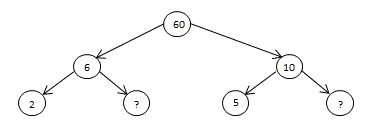Suppose the two ‘?’ are a and b
From the given factor tree we know that
60 = 6 × 10
Therefore,
6 = 2 × a and 10 = 5 × b
⇒ a = 6 ÷ 2 and b = 10 ÷ 5
⇒ a = 3 and b = 2
Therefore, the missing numbers are 3 and 2.
The prime factors of 60 = 2 × 3 × 2 × 5

(b)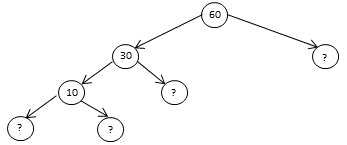Suppose the four ‘?’ are a, b, c and d
From the given factor tree we know that
60 = 30 × a and 30 = 10 × b and 10 = c × d
⇒ a = 60 ÷ 30 and b = 30 ÷ 10 and d = 10 ÷ c
⇒ a = 2 and b = 3 and c = 2; d = 5
Therefore, the missing numbers are 2, 3, 2 and 5.
The prime factors of 60 = 2 × 3 × 2 × 5

Q3. Which factors are not included in the prime factorisation of a composite number?

Prime factorization is when a composite number is broken down into the prime numbers that, when multiplied together, gives that composite number again.

• 1 is neither a prime nor a composite number.
• The numbers other than 1 whose only factors are 1 and the number itself are called Prime number.
• Numbers having more than two factors are called Composite numbers.
1 and the number itself are the factors which are not included in the prime factorisation of a composite number.

Q4. Write the greatest 4-digit number and express it in terms of its prime factors.

The greatest 4-digit number is 9999.

 3 9999 3 3333 11 1111 101 101 1

From the figure above we can say that the prime factors of 9999 are 3 × 3 × 11 × 101

Q5. Write the smallest 5-digit number and express it in the form of its prime factors.
The smallest 5-digit number is 10000.

 2 10000 2 5000 2 2500 2 1250 5 625 5 125 5 25 5

The prime factors of 10000 are 2 × 2 × 2 × 2 × 5 × 5 × 5 × 5

Q6. Find all the prime factors of 1729 and arrange them in ascending order. Now state the relation, if any; between two consecutive prime factors.

Prime factorisation for 1729

 7 1729 13 247 19 19 1

So, 1729 = 7 × 13 × 19
Therefore the prime factors of 1729 in ascending order are 7, 13 and 19.

19 - 6 = 13 and 13 - 6 = 7
So we can say that the relation between 7, 13 and 19 is that here the difference between two consecutive prime factors is equal to 6.

Q7. The product of three consecutive numbers is always divisible by 6. Verify this statement with the help of some examples.

To prove, let us take three consecutive integers, say, n, n + 1 and n + 2.

Whenever a number is divided by 3 the remainder obtained is either 0 or 1 or 2.

let n = 3p or 3p + 1 or 3p + 2, where p is some integer.

If n = 3p, then n is divisible by 3.

If n = 3p + 1, then n + 2 = 3p + 1 + 2 = 3p + 3 = 3(p + 1) is divisible by 3.

If n = 3p + 2, then n + 1 = 3p + 2 + 1 = 3p + 3 = 3(p + 1) is divisible by 3.

So that one of n, n + 1 and n + 2 is always divisible by 3.

⇒ n (n + 1) (n + 2) is divisible by 3.

Similarly, if n can either be even (divisible by 2) or odd. If n is odd, then n+1 will be even. Therefore, in three consecutive numbers at least 1 will be even i.e. divisible by 2
⇒ n (n + 1) (n + 2) is divisible by 2.
We know that, if a number is divisible by two co-prime numbers then it is divisible by their product also. So if a number is divisible by 3 and 2, it will also be divisible by 3 x 2 = 6

Examples

(a) 2 x 3 x 4 = 24, which is divisible by 6

(b) 9 × 10 × 11 = 990, which is divisible by 6
(c) 20 × 21 × 22 = 9240, which is divisible by 6
(d) 6 × 7 × 8 = 336, which is divisible by 6
(e) 4 × 5 × 6 = 120, which is divisible by 6

From the above examples it is clear that the product of three consecutive numbers is always divisible by 6

Q8. The sum of two consecutive odd numbers is divisible by 4. Verify this statement with the help of some examples.

Examples
(a) 3 + 5 = 8 and 8 is divisible by 4
(b) 5 + 7 = 12 and 12 is divisible by 4
(c) 7 + 9 = 16 and 16 is divisible by 4
(d) 9 + 11 = 20 and 20 is divisible by 4
(e) 11 + 13 = 24 and 24 is divisible by 4
(f) 21 + 23 = 44 and 44 is divisible by 4

From the above examples it is clear that the sum of two consecutive odd numbers is divisible by 4.

Q9. In which of the following expressions, prime factorisation has been done?
(a) 24 = 2 × 3 × 4 (b) 56 = 7 × 2 × 2 × 2 (c) 70 = 2 × 5 × 7 (d) 54 = 2 × 3 × 9

When a composite number is broken down into the prime numbers that, when multiplied together, gives that composite number again, we say that prime factorisation has been done.

(a) Prime factorisation for 24

 2 24 2 12 2 6 3

The prime factors for 24 = 2 × 2 × 2 × 3
Given, 24 = 2 × 3 × 4
In this case prime factorisation has not been done.

(b) Prime factorisation for 56

 2 56 2 28 2 14 7

The prime factors for 56 = 2 × 2 × 2 × 7
Given, 56 = 7 × 2 × 2 × 2
In this case prime factorisation has been done.

(c) Prime factorisation for 70

 2 70 5 35 7

The prime factors for 70 = 2 × 5 × 7
Given, 70 = 2 × 5 × 7
In this case prime factorisation has been done.

(d) Prime factorisation for 54

 2 54 3 27 3 9 3

The prime factors for 54 = 2 × 3 × 3 × 3
Given, 54 = 2 × 3 × 9
In this case prime factorisation has not been done.

Q10. Determine if 25110 is divisible by 45.
[Hint : 5 and 9 are co-prime numbers. Test the divisibility of the number by 5 and 9].

45 = 5 × 9
Divisibility by 5 : a number which has either 0 or 5 in its ones place is divisible by 5
The number 25110 has ‘0’ in ones place so it is divisible by 5.

Divisibility by 9 : if the sum of the digits of a number is divisible by 9, then the number itself is divisible by 9.
The sum of the digits = 2 + 5 + 1 + 1 + 0 = 9, which is divisible by 9

We know that, if a number is divisible by two co-prime numbers then it is divisible by their product also.
As 5 and 9 are co-primes and the number is divisible by both 5 and 9, therefore the number is divisible by their product i.e. 45.

Q11. 18 is divisible by both 2 and 3. It is also divisible by 2 × 3 = 6. Similarly, a number is divisible by both 4 and 6. Can we say that the number must also be divisible by 4 × 6 = 24? If not, give an example to justify your answer.

No, a number that is divisible by both 4 and 6 is not always divisible by its product i.e. 4 × 6 = 24, because 4 and 6 are not co-primes
Examples:
12 is divisible by both 4 and 6 but not divisible by 24
36 is divisible by both 4 and 6 but not divisible by 24
60 is divisible by both 4 and 6 but not divisible by 24

Q12. I am the smallest number, having four different prime factors. Can you find me?

The first 4 prime numbers are 2, 3, 5 and 7.
2 × 3 × 5 × 7 = 210
Hence, 210 is the smallest number having four different prime factors.

Page 63

Exercise 3.6

Q1. Find the HCF of the following numbers:
(a) 18, 48 (b) 30, 42 (c) 18, 60 (d) 27, 63 (e) 36, 84 (f) 34, 102 (g) 70, 105, 175
(h) 91, 112, 49 (i) 18, 54, 81 (j) 12, 45, 75
(a) 18, 48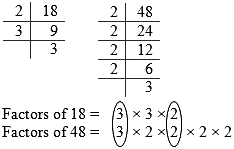HCF (18, 48) = 3 × 2 = 6

(b) 30, 42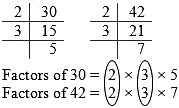H.C.F. (30, 42) = 2 × 3 = 6

(c) 18, 60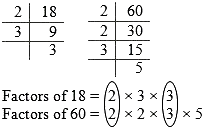HCF (18, 60) = 2 × 3 = 6

(d) 27, 63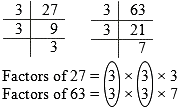HCF (27, 63) = 3 × 3 = 9

(e) 36, 84HCF (36, 84) = 2 × 2 × 3 = 12

(f) 34, 102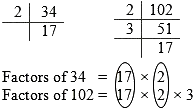HCF (34, 102) = 17 × 2 = 34

(g) 70, 105, 175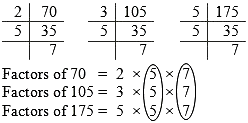HCF (34, 102, 175) = 5 × 7 = 35

(h) 91, 112, 49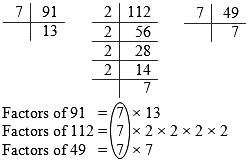HCF (70, 112, 49) = 7

(i) 18, 54, 81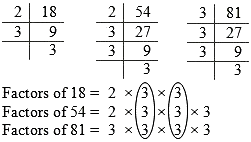HCF (18, 54, 81) = 3 × 3 = 9

(j) 12, 45, 75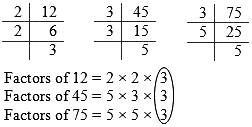HCF (12, 45, 75) = 3

Q2. What is the HCF of two consecutive (a) numbers? (b) even numbers? (c) odd numbers?
(a) Let us consider two sets of consecutive numbers -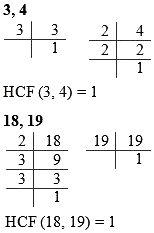Hence we can say that the HCF of two consecutive numbers is 1.

(b) Let us consider two sets of consecutive even numbers -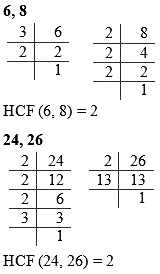Hence we can say that the HCF of two consecutive even numbers is 2.

(c) Let us consider two sets of consecutive odd numbers -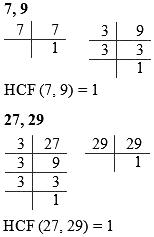Hence we can say that the HCF of two consecutive odd numbers is 1.

Q3. HCF of co-prime numbers 4 and 15 was found as follows by factorisation :
4 = 2 × 2 and 15 = 3 × 5
since there is no common prime factor, so HCF of 4 and 15 is 0. Is the answer correct? If not, what is the correct HCF?

No, HCF of 4 and 15 is 0 is the wrong answer.

Factorisation of 4 and 15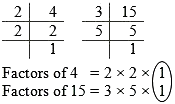HCF (4, 15) = 1

Co-prime numbers have only 1 as common factor, so HCF of 4 and 15 will be 1.

Page 67

Exercise 3.7

Q1. Renu purchases two bags of fertiliser of weights 75 kg and 69 kg. Find the maximum value of weight which can measure the weight of the fertiliser exact number of times.

To find maximum weight, we will have to find HCF of 75 and 69.
Factorisation of 75 and 69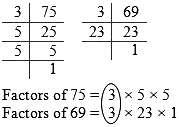Therefore, the maximum value of weight which can measure the weight of the fertiliser exact number of times is 3 kgs.

Q2. Three boys step off together from the same spot. Their steps measure 63 cm, 70 cm and 77 cm respectively. What is the minimum distance each should cover so that all can cover the distance in complete steps?

To find the minimum distance, we have to find LCM of 63, 70 and 77.

LCM of 63, 70 and 77

 2 63     70     77 3 63     35     77 3 21     35     77 5 7     35     77 7 7        7     77 11 1        1     11 1        1        1

LCM of 63, 70 and 77 = 11 × 5 × 2 × 3 × 3 × 7
= 7 × 11 × 10 × 9
= 63 × 110
= 6930

Therefore, the minimum distance each should cover so that all can cover the distance in complete steps is 6930 cms.

Q3. The length, breadth and height of a room are 825 cm, 675 cm and 450 cm respectively. Find the longest tape which can measure the three dimensions of the room exactly.

To find the longest tape, we have to find HCF of 825 cm, 675 cm and 450 cm.

Factorisation of 825, 675 and 450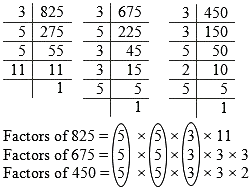HCF of 825, 675 and 450 = 5 × 5 × 3
= 75

Therefore, the longest tape which can measure the three dimensions of the room exactly will be 75 cms.

Q4. Determine the smallest 3-digit number which is exactly divisible by 6, 8 and 12.

To find the smallest 3-digit number we will first find the LCM of the given numbers 6, 8 and 12.

Factorisation of 6, 8 and 12

 2 6     8     12 2 3      4       6 2 3      2       3 3 3      1       3 1      1       1

LCM of 6, 8 and 12 = 2 × 3 × 2 × 2
= 24

Now we need to find the smallest 3-digit multiple of 24.
24 × 3 = 72
24 × 4 = 96
24 × 5 = 120

120 is the smallest three-digit multiple of 24.
24 + 6 = 4
24 + 8 = 3
24 + 12 = 2

Therefore, the smallest 3-digit number which is exactly divisible by 6, 8 and 12 is 120.

Q5. Determine the greatest 3-digit number exactly divisible by 8, 10 and 12.

To find the smallest 3-digit number we will first find the LCM of the given numbers 8, 10 and 12.

Factorisation of 8, 10 and 12

 2 8     10     12 2 4        5        6 2 2        5        3 3 1        3        5 5 1        1        5 1        1        1

LCM of 8, 10 and 12 = 2 × 2 × 2 × 3 × 5
= 120

Now we need to find the greatest 3-digit multiple of 120.
120 × 5 = 600
120 × 6 = 720
120 × 7 = 840
120 × 8 = 960
120 × 9 = 1080

960 is the greatest three-digit multiple of 120.
960 ÷ 8 = 120
960 ÷ 10 = 96
960 ÷ 12 = 80

Therefore, the greatest 3-digit number exactly divisible by 8, 10 and 12 is 960.

Q6. The traffic lights at three different road crossings change after every 48 seconds, 72 seconds and 108 seconds respectively. If they change simultaneously at 7 a.m., at what time will they change simultaneously again?

The Lowest Common Multiple (LCM) of their changing times will give the time when the traffic lights will change simultaneously again. To find LCM we will first find the prime factorisations of 48, 72 and 108.

 2 48      72     108 2 24      36        54 2 12      18        27 2 6         9        27 3 3         9        27 3 1         3           9 3 1         1           3 1         1           1

LCM of 48, 72 and 108 = 2 × 2 × 2 × 2 × 3 × 3 × 3
= 432

So we can say that after 432 seconds, the lights will change simultaneously.

432 second = 432 + 60
= 7.2 minutes or
= 7 minutes and (.2 × 60) seconds
= 7 minutes 12 seconds

Therefore, the time when the traffic lights will change simultaneously again will be
= 7 am + 7 min 12 sec
= 07:07:12 am

Q7. Three tankers contain 403 litres, 434 litres and 465 litres of diesel respectively. Find the maximum capacity of a container that can measure the diesel of the three containers exact number of times.

The maximum capacity of a container that can measure the diesel of the three containers exact number of times will be the HCF of the given capacities of the three tankers.

We find the HCF of 403, 434 and 465 by factorisation of 403, 434 and 465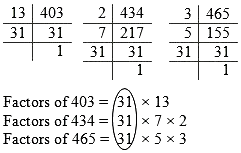HCF of 403, 434 and 465 = 31

Therefore, the maximum capacity of a container that can measure the diesel of the three containers exact number of times will be 31 litres.

Q8. Find the least number which when divided by 6, 15 and 18 leave remainder 5 in each case.

To find the least number which when divided by 6, 15 and 18 leave remainder 5 in each case, first we will find LCM of 6, 15 and 18.

 2 6      15      18 3 3      15         9 3 1         5         3 5 1         5         1 1         1         1

LCM of 6, 15 and 18 = 2 × 3 × 3 × 5 = 90

90 is the least number which will have 0 as remainder when divided by the numbers 6, 15 and 18.
In the given question, we need the least number that leaves remainder 5 in each case. Therefore, the required number will be 5 more than 90.
90 + 5 = 95

The least number which when divided by 6, 15 and 18 and will leave a remainder of 5 in each case will be 95.

Q9. Find the smallest 4-digit number which is divisible by 18, 24 and 32.

To find the smallest 4-digit number which is divisible by 18, 24 and 32, we will find LCM of these numbers.
LCM of 18, 24 and 32

 2 18     24     32 2 9     12     16 2 9        6        8 2 9        3        4 2 9        3        2 3 9        3        1 3 3        1        1 1        1        1

LCM of 18, 24 and 32 = 2 × 2 × 2 × 2 × 2 × 3 × 3
= 288

Now we need to find the smallest 4-digit multiple of 288.

288 × 2 = 576
288 × 3 = 864
288 × 4 = 1152
288 × 5 = 1440

1152 is the smallest 4-digit multiple of 288.
1152 ÷ 18 = 64
1152 ÷ 24 = 48
1152 ÷ 32 = 36

Therefore, the smallest 4-digit number which is divisible by 18, 24 and 32 is 1152.

Q10. Find the LCM of the following numbers :
(a) 9 and 4 (b) 12 and 5 (c) 6 and 5 (d) 15 and 4
Observe a common property in the obtained LCMs. Is LCM the product of two numbers in each case?

(a) LCM of 9 and 4

 2 9     4 2 9     2 3 9     1 3 3     1 1     1

LCM of 9 and 4 = 2 × 2 × 3 × 3
= 36

(b) LCM of 12 and 5

 2 12     5 2 6     5 3 3     5 5 1     5 1     1

LCM of 12 and 5 = 2 × 2 × 3 × 5
= 60

(c) LCM of 6 and 5

 2 6     5 3 3     5 5 1     5 1     1

LCM of 6 and 5 = 2 × 3 × 5
= 30

(d) LCM of 15 and 4

 2 15     4 2 15     2 3 15     1 5 5     1 1     1

LCM of 15 and 4 = 2 × 2 × 3 × 5
= 60

We can observe in the given cases that when the numbers are co-primes, the LCM of the numbers is their product.
Yes, the L.C.M. is equal to the product of two numbers in each case.

Q11. Find the LCM of the following numbers in which one number is the factor of the other.
(a) 5, 20 (b) 6, 18 (c) 12, 48 (d) 9, 45
What do you observe in the results obtained?

(a) LCM of 5 and 20

 2 5     20 2 5     10 5 5        5 1        1

LCM of 6 and 5 = 2 × 2 × 5
= 20

(b) LCM of 6 and 18

 2 6     18 3 3        9 3 1        3 1        1

LCM of 6 and 18 = 2 × 3 × 3
= 18

(c) LCM of 12 and 48

 2 12     48 2 6     24 2 3     12 2 3        6 3 3        3 1        1

LCM of 12 and 48 = 2 × 2 × 2 × 2 × 3
= 48

(d) LCM of 9 and 45

 3 9     45 3 3     15 5 1        5 1        1

LCM of 9 and 45 = 3 × 3 × 5
= 45

From the given cases, we can conclude that if the smaller number is the factor of larger number, then the LCM of these two numbers is equal to the larger number.